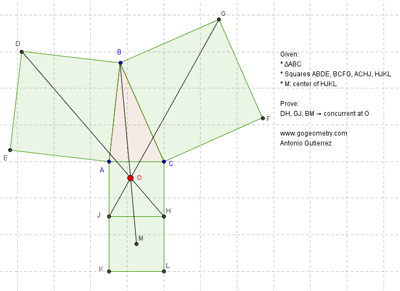Monday, July 15, 2013

Problem 902: Triangle, Four Squares, Center, Concurrent Lines

Geometry Problem. GeoGebra, HTML5 Animation for iPad and Nexus.
Post your solution in the comments box below.

Level: Mathematics Education, High School, Honors Geometry, College.

Click here to see the static diagram of problem 902.
Click here to see the dynamic diagram of problem 902.1 comment:

1.Connect BJ and EC, which intersect perpendicularly at V; connect BH and AF, which intersect perpendicularly at W (Vecten theorem). Connect VW. Draw circle ACHJ, which passes through V and W (because of right angles at V and W). Connect HM and JM, which are tangents to circle ACHJ. Then cyclic quadrilateral VWHJ with tangents at H and J becomes a 4-point degenerate case of Pascal's hexagon theorem. Thus BM, VH and WJ are concurrent at O. QED